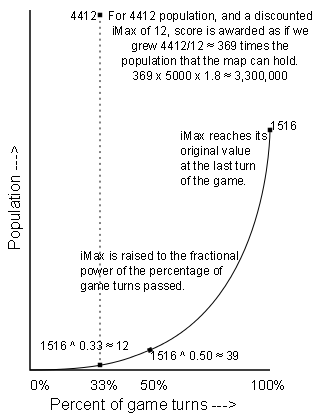### Civilization 4 Victory Score Calculator

See my main article for an explanation.

Here is a Javascript implementation of the normalized score formula. You can use this to simulate score scenarios by entering values from a real or hypothetical game. A great and common question is, how much will my victory score drop for each new turn? Now we can answer that! The pre-populated values are from my huge map marathon Persian Sushi game. The calculations here come out accurate to the penny with no roundoff error; I think this is the first time this has been accomplished outside of the game itself.

To use it, enter your turn and speed and difficulty, and fill in the first two columns in the table. iRawScore and iMax are the two numbers shown for each component in-game when you mouse over your name on the scoreboard, such as 5/310 for wonders.

Notice how population dominates the other components by a huge margin, and wonders score almost nothing.

Turn: Speed: Difficulty:

iRawScore iMax iInitial iFactor fRatio fTurnRatio new iMax iScore Difficulty Component Score
Total Score:

(I've rounded the columns to a few decimal places for display purposes, but the calculations use full precision. In the formula, there is integer truncation only for fRatio and the last Component Score number, no other values are truncated or rounded at all.)Update 3/20/2012: I've changed the demonstration values to those from my Persia marathon game using Sid's Sushi.

I'm also going to take a few words to explain the "new iMax" column, since that's the linchpin of how the score works. "New iMax" is the discounted value of the original maximum. When the scoreboard says 4412/1516 population, the new iMax is the result of exponentially discounting that 1516 max. We take 1516 to the power of fTurnRatio, so 1516 ^ 0.3387 = 11.94. The idea of this operation is that if the civ were to grow from 1 pop on turn 1 to 1516 pop on turn 1500, always at the same exponential rate, the civ would have 11.94 population on turn 508.

So the population score is calculated as if the civ got 4412 population on a map where the "maximum" population was 11.94. That's why these scores go so crazy into multiple millions. Credit is awarded as if you built 4412/11.94 = 369 times more population than the map can hold! Multiply 369 x 5000 (weight factor for population) x 1.8 (for Immortal) and that comes to the 3,300,000 population score.

That's also why bigger maps are better. Suppose you double the original iMax by moving to a bigger map. The discounted iMax changes by much less than double! 1500 ^ 0.34 = 12, but 3000 ^ 0.34 = 15. So if you double both the population and the map size, you get 2 * 12/15 = 1.6 times as much score! (That 1.6 figure for doubling both pop and size is not constant, it depends on the game turns passed, but holds close to constant around the sweet spot of turn 500 on marathon.)

Also available is an Excel implementation.